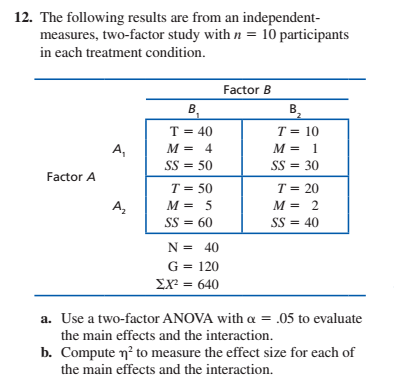# 12. The following results are from an independent-measures, two-factor study with n = 10 participantsin each treatment condition.Factor BB.B,T = 40T = 10%3!%3!A,M = 4M = 1SS = 50SS = 30Factor AT = 50T = 20M = 5M = 2A,SS = 40SS = 60N = 40G = 120EX = 640a. Use a two-factor ANOVA with a = .05 to evaluatethe main effects and the interaction.b. Compute n? to measure the effect size for each ofthe main effects and the interaction.

Question
30 viewshelp_outlineImage Transcriptionclose12. The following results are from an independent- measures, two-factor study with n = 10 participants in each treatment condition. Factor B B. B, T = 40 T = 10 %3! %3! A, M = 4 M = 1 SS = 50 SS = 30 Factor A T = 50 T = 20 M = 5 M = 2 A, SS = 40 SS = 60 N = 40 G = 120 EX = 640 a. Use a two-factor ANOVA with a = .05 to evaluate the main effects and the interaction. b. Compute n? to measure the effect size for each of the main effects and the interaction. fullscreen
check_circle

Step 1

(a) The data is provided for an independent measures, two-factor study with n=10 participants in each treatment condition.

The level of significance is provided as 0.05. Using the provided data, the degrees of freedom can be calculated as:

Step 2

The sum of squares can be calculated as:

Step 3

The mean sum of squares c...

### Want to see the full answer?

See Solution

#### Want to see this answer and more?

Solutions are written by subject experts who are available 24/7. Questions are typically answered within 1 hour.*

See Solution
*Response times may vary by subject and question.
Tagged in

### Statistics